Reflections
Rotations
Translations
Name that transformation
Congruence
Dilations
100

Reflect (2, 3) over the x-axis

(2, -3)

100

Rotate (2, 3) 90 degrees

(-3, 2)

100

Translate (2,3) to 2 units right

(4, 3)

100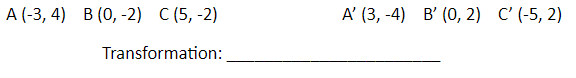180 degree rotation

100

Determine whether the polygons with the given vertices are congruent. If so, describe the transformations to prove it.

Q(2, 4), R(5, 4), S(4, 1)

T(6, 4), U(9, 4), V(8, 1)

Yes, triangle TUV is a translation 4 units right of triangle QRS.

100

Dilate (-3,2) by a scale factor of k=2

(-6,4)

200

Reflect (0, 9) over the x-axis

(0, -9)

200

Rotate (-3, 2) 270 degrees counter clockwise

(2, 3)

200

Translate (2, 3) to 4 units up

(2, 7)

200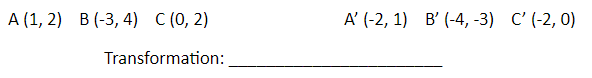90 degree rotation counterclockwise

200

Which transformations are rigid motions?

Reflections, Translations, Rotations

200

Find the scale factor. Then determine if its an enlargement or reduction.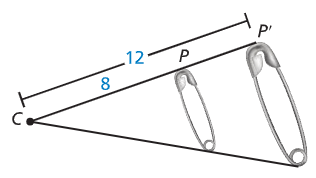3/2 Enlargement

300

Reflect (6, -4) over the y-axis

(-6, -4)

300

Rotate (6, -4) 180 degrees

(-6, 4)

300

Translate (4, 2) to 3 units left, 1 unit down

(1, 1)

300translation 4 left and 7 down

300

Find the angle of rotation that maps A onto A''.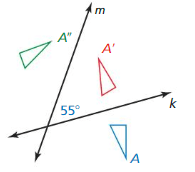110 degrees

300

Find the scale factor. Then determine if its an enlargement or reduction.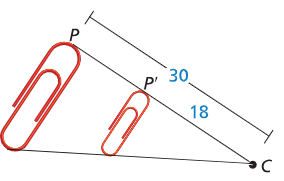3/5 reduction

400

Reflect (-2, 3) over the line y=x.

(3, -2)

400

(0, 9) transforms to (-9, 0). Identify the rotation.

90 degrees counterclockwise

400

Translate (3, 1) to 5 units right, 5 units up

(8, 6)

400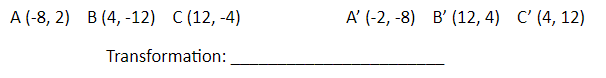rotation 90 degrees counterclockwise

400

Describe a congruence transformation that maps the blue preimage to the green image.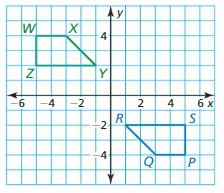180 degree rotation about the origin

400

The image of a spider can be seen through a magnifying glass that is 6 times larger than its actual size. Find the actual size of the spider.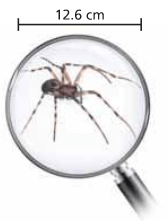2.1cm

500

Reflect (-4, -1) over the line y = -x.

(1, 4)

500

(-4, -4) turns into (4, 4). Identify the rotation.

180 degrees

500

Translate (-7, -1) to 3 units left, 1 unit up

(-10, 0)

500

what combination of transformations was used to get from ABC to A'B'C'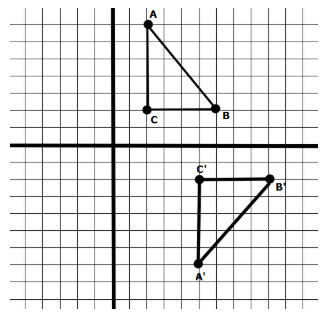3 to the right, reflect over x-axis

500

Describe a congruence transformation that maps the blue preimage to the green image.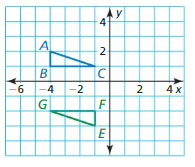180 rotation about the origin followed by a translation 5 units left and 1 unit down

500

What are the dimensions of a 3in x 5in photo that is enlarged by 125%?

3.75in x 6.25in

Click to zoom